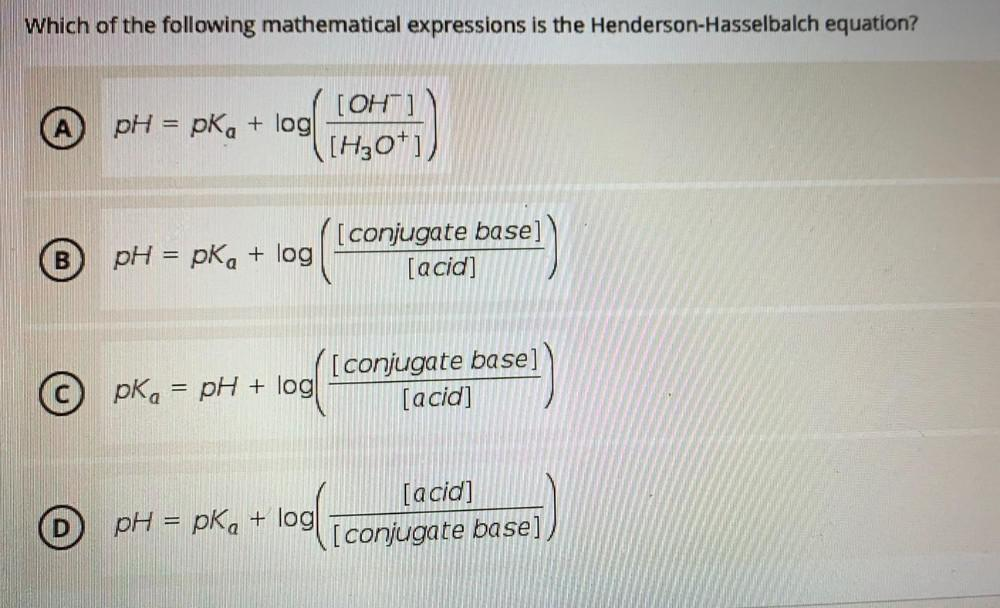Question:

# Which of the following mathematical expressions is the

Last updated: 7/28/2022Which of the following mathematical expressions is the Henderson-Hasselbalch equation? (A) pH = pka + log(]OH‾]/[H3O⁺]) (B) pH = pka + log([conjugate base]/[acid]) (C) pka = pH + log([conjugate base]/[acid]) (D) pH = pka + log([conjugate base]/[acid])